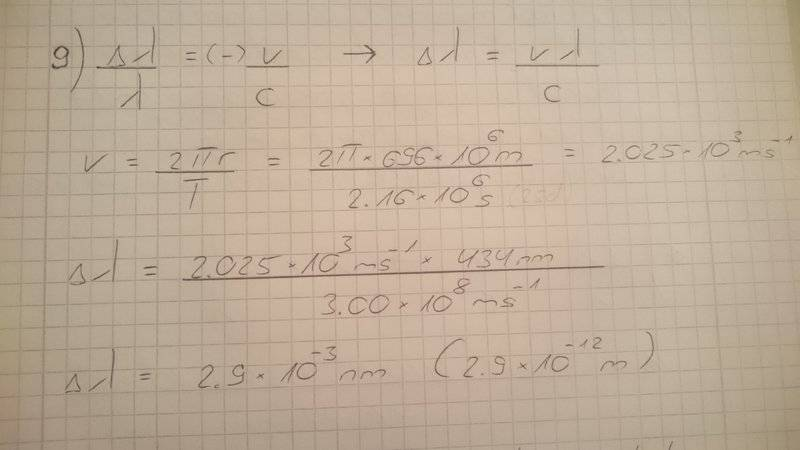# Doppler shift of a hydrogen line on sun

Krashy

## Homework Statement## Homework Equations

[/B]
delta lambda/lambda = velocity/speed of light

radius of the sun= 696 *10^6m

## The Attempt at a SolutionHello,
the solution states the correct answer for this problem is 5.8*10^-12m. This is exactly 2x my answer but i dont really know what i did wrong. There is also a possibility that the solution is wrong because there are quite a few cases in which it is wrong so i cant be 100% sure.
So i hope someone can tell me where i messed up. Thanks for every answer.

#### Attachments

Last edited:

Staff Emeritus
Homework Helper
Gold Member
2021 Award
radius of earth = 696 *10^6m
You mean the radius of the Sun.

This is exactly 2x my answer but i dont really know what i did wrong.
What is your ##\Delta \lambda## the wavelength difference between? Is this the wavelength difference you are interested in?

Krashy
Oh yeah sorry i meant the radius of the sun. I am searching for the change in wavelength i think and so the shift between blue and red should be 2.9*10^-12m, right?

Staff Emeritus
Homework Helper
Gold Member
2021 Award
I am searching for the change in wavelength i think and so the shift between blue and red should be 2.9*10^-12m, right?
No, think a bit about what you are computing the wavelength shift relative to. What is the original wavelength the wavelength of and what is the shifted wavelength the wavelength of?

Krashy
I dont think i really get it. As the sides of the sun move away and towards the earth, the spectral line splits into two, right? So the "real" wavelength is 434nm and the shift towards either side should be +/- 2.9*10^-12m, because the doppler shifts are the same only in opposite directions, or is that wrong?

Staff Emeritus
Homework Helper
Gold Member
2021 Award
As the sides of the sun move away and towards the earth, the spectral line splits into two, right? So the "real" wavelength is 434nm and the shift towards either side should be +/- 2.9*10^-12m, because the doppler shifts are the same only in opposite directions, or is that wrong?
No, that is correct. So what is the difference between the wavelengths at the edges in opposite directions? (Which is what the problem is asking for.)

•Krashy
Krashy
All right i think i understand now. The change in wavelength from one side to the middle, so to the "real" wavelength is 2.9*10^-12m. Thus the shift from one side to the other side is 2x that value: 2 * 2.9*10^-12m = 5.8 *10^-12m. This should be correct now, right? Thank you very much for the clarification.

Staff Emeritus